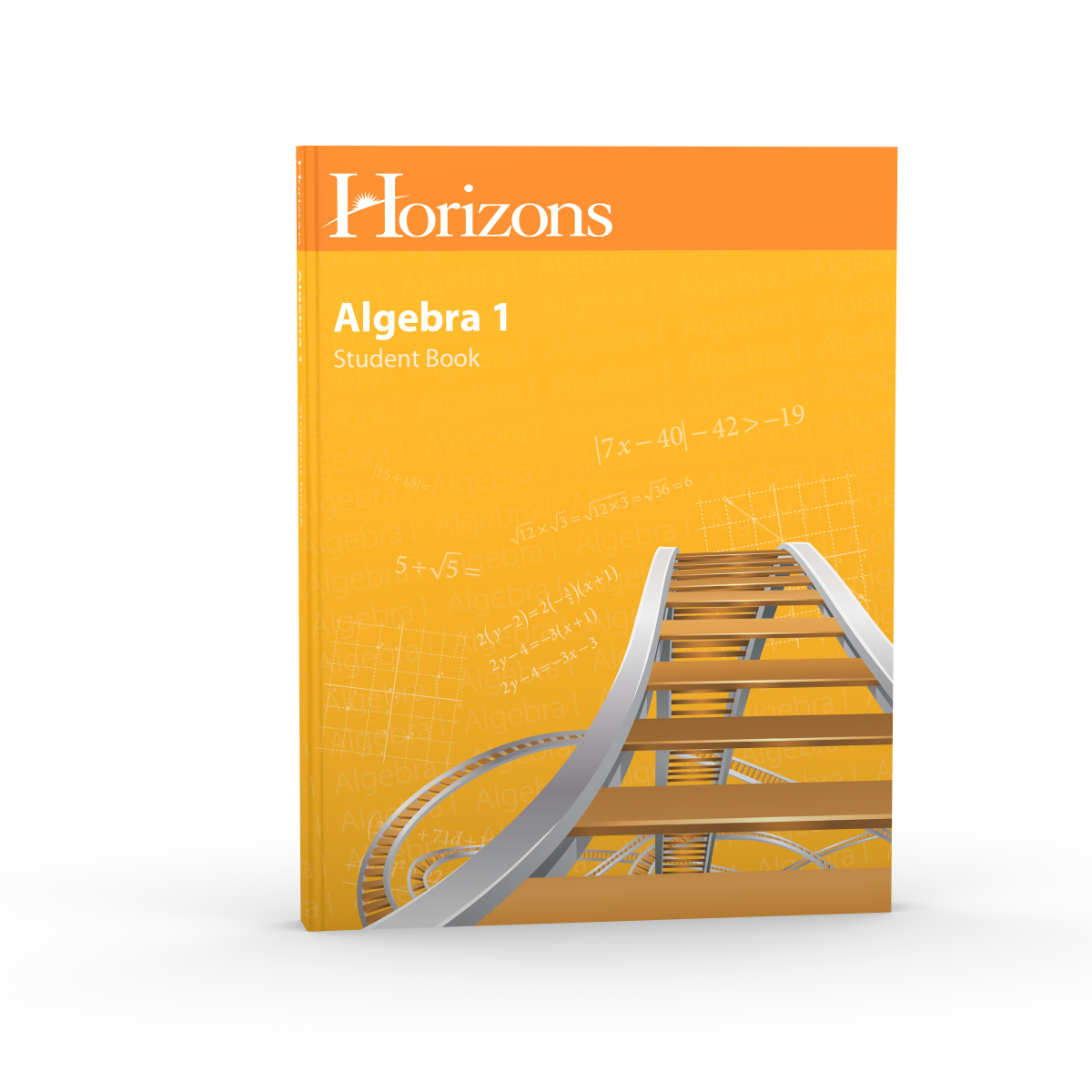# Horizons Algebra I Student Book

Product Id - JMS081## Description

Explore the world of algebraic concepts with the Horizons Algebra I Student Book! Making advanced math more appealing and easier with eye-catching illustrations and an easy-to-follow lesson format, this colorful Alpha Omega curriculum reviews factoring, prime numbers, scientific notation, and algebraic expressions, while introducing your child to systems of equations, rational expressions, systems of linear equations, basic operations with monomials and polynomials, quadratic equations with radicals, graphs of exponential functions, and more.

Each teacher-directed Horizons Math lesson in the student book includes a teaching box that outlines the new concept being introduced, a classwork section that reinforces the information with teacher-guided practice questions, and an activities section with problems that cover the current lesson topic and concepts from previous lessons. The Horizons Algebra I Student Book also prepares your child for standardized testing with a set of college test prep questions that follows each block of ten lessons. Plus, the student book contains 16 sports and real-life applications that give more meaning to math.